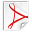# Least squares with partition of unity constraint

November 2014You can also download this derivation in PDF form.

## Unconstrained solution

Suppose that you have an unconstrained least squares problem, where the error function is written in matrix form as: $$E(x) = x^T A x - 2 x^T B + C$$ The minimizer is found by solving for where the gradient of the error $E(x)$ is equal to zero. $$\frac {dE(x)} {dx} = 2Ax - 2B = 0$$ The minimum is therefore found by solving the system $$Ax = B.$$

## Partition of unity constraint

It is sometimes necessary to solve a least squares system under the constraint that the sum of coefficients is equal to one. This is called a partition of unity. $$\sum_{i=1}^n x_i = 1$$ One way to add this constraint is to notice that the constraint is linear, and can therefore be enforced using Lagrange multipliers. I will not go into details of that approach, because it is bad for this problem. Lagrange multipliers increase the size of the system by one for each linear constraint and make the system poorly conditioned. For partition of unity, we can instead reduce the size of the system by one.

We can split the coefficient vector into an unconstrained part $x$ and a constrained part $y$ that depends on $x$. $$x = \begin{pmatrix} x_1 \\ x_2 \\ \vdots \\ x_{n-1} \end{pmatrix}$$ $$N = \begin{pmatrix}1 & 1 & \ldots & 1\end{pmatrix}$$ $$y = 1 - Nx$$ Rewriting the error function in block form, we get $$E(x) = \begin{pmatrix}x^T & y^T\end{pmatrix} \begin{pmatrix}A_{xx} & A_{xy} \\ A_{yx} & A_{yy} \end{pmatrix}\begin{pmatrix}x \\ y\end{pmatrix} - 2 \begin{pmatrix}B_x & B_y\end{pmatrix} \begin{pmatrix}x \\ y\end{pmatrix} + C.$$ This expands to \begin{align*} E(x) & = x^T A_{xx} x \\ & + x^T A_{xy} y + y^T A_{yx} x \\ & + y^T A_{yy} y \\ & - 2 x^T B_x - 2 y^T B_y + C. \end{align*} Substituting $y = 1 + Nx$ gives \begin{align*} E(x) & = x^T A_{xx} x \\ & + x^T A_{xy} (1 - Nx) + (1 - x^T N^T) A_{yx} x \\ & + (1 - x^T N^T) A_{yy} (1 - Nx) \\ & - 2 x^T B_x - 2 (1 - x^T N^T) B_y + C. \end{align*} Expanding again: \begin{align*} E(x) & = x^T A_{xx} x \\ & + 2 x^T A_{xy} - x^T A_{xy} Nx - x^T N^T A_{yx} x \\ & + A_{yy} - 2 x^T N^T A_{yy} + x^T N^T A_{yy} Nx \\ & - 2 x^T B_x - 2 B_y + 2 x^T N^T B_y + C. \end{align*} Taking the derivative w.r.t. $x$ \begin{align*} \frac {dE(x)}{dx} & = 2 A_{xx} x \\ & + 2 A_{xy} - 2 A_{xy} Nx - 2 N^T A_{yx} x \\ & - 2 N^T A_{yy} + 2 N^T A_{yy} Nx \\ & - 2 B_x + 2 N^T B_y. \end{align*} As before, we want to solve for when $\frac {dE(x)}{dx} = 0$ $$2 A_{xx} x + 2 A_{xy} - 2 A_{xy} Nx - 2 N^T A_{yx} x - 2 N^T A_{yy} + 2 N^T A_{yy} Nx - 2 B_x + 2 N^T B_y = 0$$ This can be written in the form of $Ax=B$ as: $$(A_{xx} - A_{xy} N - N^T A_{yx} + N^T A_{yy} N) x = B_x - A_{xy} + N^T A_{yy} - N^T B_y.$$